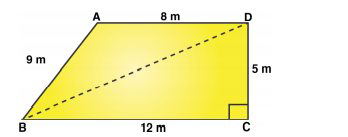Rd sharma solutionsOur top 5% students will be awarded a special scholarship to Lido.
Set your child up for success with Lido, book a class today!

# Question 1

A park in the shape of a quadrilateral ABCD, has ∠ C =90^0, AB = 9 m, BC = 12 m, CD = 5 m, AD = 8 m. How much area does it occupy?

• Solution

• TranscriptHere, AB = 9 m, BC = 12 m, CD = 5 m, DA = 8 m.

And BD is a diagonal of ABCD.

In right △BCD,

From Pythagoras theorem;

BD2 = BC2 + CD2

BD2 = 122 + 52 = 144 + 25 = 169

BD = 13 m

Area of △BCD = 1/2×BC×CD

= 1/2×12×5

Area of △BCD = 30

m^2

Now, In △ABD,

All sides are known, Apply Heron’s Formula:"hello students my name is megha and i am a tutor at leader learning in this q a video we will explore the concept of heron's formula question a park in the shape of recta quadrilateral abcd has angle c equals to 90 degree angle b a b is equals to 9 bc 12 cd5 88 how much area does it occupy firstly let's make a diagram based on the instructions that are given to us let's draw the diagram based on the figure given to us so this is the diagram we have given to us okay now give values put naming a b c d now put the values 8 5 12 9 and this we have given this is a right angle at angle c now what you have to do you have to find the area of this coordinated park what you have to do you have to apply different formulas to find the quad area of triangle this and to find the area of triangle this you know this triangle b c d is a right angle triangle and we can directly apply half into base into height but to find the value of b d we have to use the pythagoras theorem in to find the value of bd so that we can find the value of triangle abd by heron's formula right so let's find the hypotenuse so hypotenuse square equals to perpendicular square plus b square which is equal to perpendicular is given 12 square plus 5 square 12 square plus 5 square is equal to 144 plus 25 which is equals to 169 right 169 therefore hypotenuse will be equals to 13 meter hypotenuse bd will be equals to 13 meter now we have bd now apply the formula of triangle to find the value of area of triangle b c d half into base into height so what is base and what is height base is 12 height is 5 so here you will get 30 square meter as area of triangle bcd now we have to find the area of triangle abd and how do we find the area of triangle abd by applying the heron's formula and what is heron's formula firstly area of triangle area of triangle abd how do we find by using the heron's formula heron's formula is semi-parameter into semi-parameter minus a semi-parameter minus b semi-parameter minus c what is semi-parameter semi-parameter is parameter divided by 2 and parameter we have 9 plus 8 plus 13 divided by 2 here we have 13 and 8 21 and 9 21 and 9 is 21 and 9 is 30 so 30 divided by 2 we have semi parameter is equals to 15 meter now apply the formula apply the values in the formula here we have semi parameter is 15 15 minus a means 15 minus 13 15 minus 13 is 2 15 minus 8 is 7 and 15 minus 9 is 15 minus 9 is 6. now we know what is the value let's make factors and get the value of this 3 into 5 15 2 7 and 3 into 2 is 6. now let's see pairing up 3 let's take 3 outside the bracket outside the root 2 outside the root and inside the root we have 5 into 7 which is equals to 7 into 5 35 so we have 6 root 35 after solving the root you will get the value of triangle abd as 35 [Applause] meter square right now add the values both the values and you will get the answer what you have to add 39 35 plus 35.49 plus 30 which is equal to 65.5 meter square approximately 49 is 5. so if you have any query in this question please drop a comment below and subscribe to our channel for more updates thank you "

Our top 5% students will be awarded a special scholarship to Lido.Lido

Courses

Teachers

Book a Demo with us

Syllabus

Science
English
Coding

Terms & Policies

NCERT Question Bank

Maths
Science

Selina Question Bank

Maths
Physics
Biology

Allied Question Bank

Chemistry